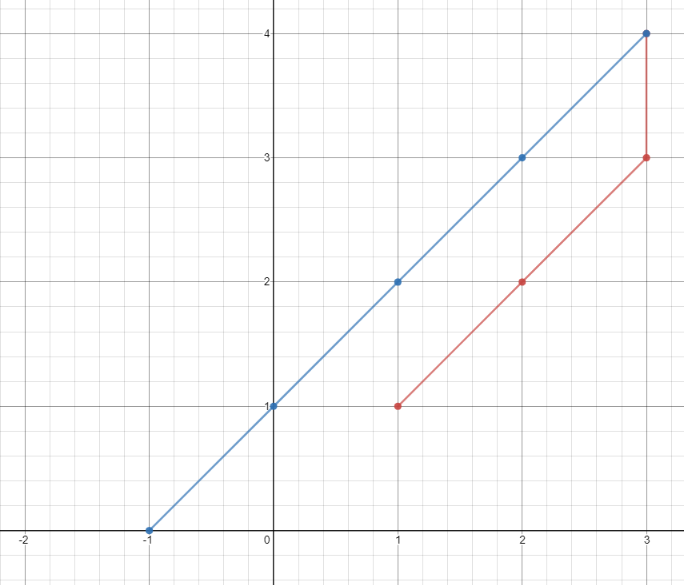# Minimum Time Visiting All Points

Easy
2
25.0% Acceptance

In this lab, you'll have to find the minimum time in seconds to visit all the given points in a 2D plane with integer coordinates. There are `n` points with integer coordinates `points[i] = [xi, yi]`. You are required to calculate the minimum time in seconds to visit all the points in the order given by `points`.

You can move according to these rules:

• In `1` second, you can either:
• move vertically by one unit,
• move horizontally by one unit, or
• move diagonally `sqrt(2)` units (in other words, move one unit vertically then one unit horizontally in `1` second).
• You have to visit the points in the same order as they appear in the array.
• You are allowed to pass through points that appear later in the order, but these do not count as visits.

## Example 1:Input: points = [[1,1],[3,4],[-1,0]] Output: 7 Explanation: One optimal path is [1,1] -> [2,2] -> [3,3] -> [3,4] -> [2,3] -> [1,2] -> [0,1] -> [-1,0]
Time from [1,1] to [3,4] = 3 seconds Time from [3,4] to [-1,0] = 4 seconds Total time = 7 seconds

## Example 2:

Input: points = [[3,2],[-2,2]] Output: 5

Constraints:

• `points.length == n`
• `1 <= n <= 100`
• `points[i].length == 2`
• `-1000 <= points[i], points[i] <= 1000`

In this lab, you will be given an initial file named `index.js` with the following code:

``````/**
* @param {number[][]} points
* @return {number}
*/
var minTimeToVisitAllPoints = function(points) {

};

export default minTimeToVisitAllPoints;``````

You need to implement the function `minTimeToVisitAllPoints` and export it, so it can be tested by the evaluation script.

There will be individual challenges related to this lab that will test your solution step by step. Make sure to test your code as you progress through the challenges.